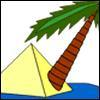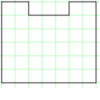#### You may also like### Triangle Island

You have pitched your tent (the red triangle) on an island. Can you move it to the position shown by the purple triangle making sure you obey the rules?### Triangle Transformation

Start with a triangle. Can you cut it up to make a rectangle?### Rod Area

This task challenges you to create symmetrical U shapes out of rods and find their areas.

# Uncanny Triangles

##### Age 7 to 11Challenge Level

Julian from Hastingsbury Upper School approached this problem using algebra:

Assuming that all the triangles are right-angled, and the two shorter sides are a and b,
Sum of two sides = a+b Area = (ab)/2
If the two are equal, then a+b = (ab)/2
Since the numbers involved are only small integers, substitute in different possible values for b. Rearrange to find the matching a. If a falls in the given range, then that makes a correct pair.
For example:
let b=1
a+1 = a/2
2a+2 = a
2a = a-2
a = -2
-2 isn't in the range of 1 to 15, so this isn't a correct pair.
The first pair is at b=3:
a+3 = (3a)/2
2a+6 = 3a
6 =a
Therefore the first pair is a=3, b=6, and the sum/area is 9.
The second pair is at b=4:
a+4 = (4a)/2
2a+8 = 4a
8 = 2a
a = 4
So the second pair is a=4, b=4, and the sum/area is 8.
One other result is when b=6, but of course this is the same as the one above.

Thank you also to Esther who sent in a correct solution although you didn't explain how you worked it out, Esther. Has anybody found another way to solve this problem other than using algebra? Let us know if you have.